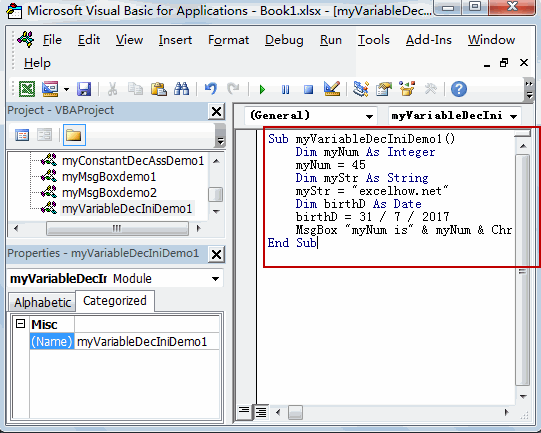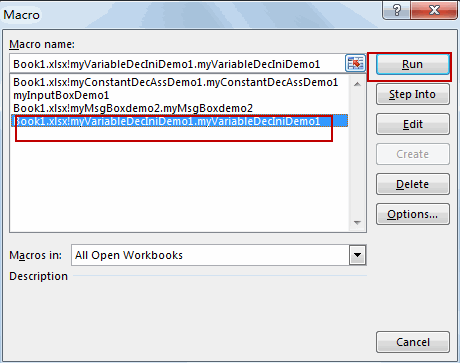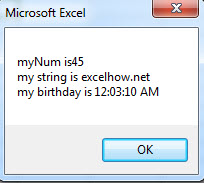# Excel VBA Variables

This post will guide you how to declare, initialize and display a variable value in MS excel VBA.

### Declare a VBA variable

If you want to declare a variable as Integer, just type the below code line:

`Dim  myNum as Integer`

Or

`Dim myNum%`

The above code declare a variable “myNum” as integer type.

### Initialize a VBA variable

It’s very simple to initialize a VBA variable, just following the below code:

```Dim myNum as Integer
myNum = 28```

You must to declare the variable firstly, then initialize its value.

### Data types

The data types contains Byte, Boolean, Integer, Long, Currency, Decimal, Single, Double, Date, String, Object, Variant and user defined type.  Each data type have different range of values, see below table:

 Type Range of Values Byte 0 to 255 Boolean True or False Integer -32768 to 32767 Long -2,147,483,648 to 2,147,483,648 Single -3.402823E+38 to -1.401298E-45 for negative values 1.401298E-45 to 3.402823E+38 for positive values. Double -1.79769313486232e+308 to -4.94065645841247E-324 for negative values 4.94065645841247E-324 to 1.79769313486232e+308 for positive values. Currency -922,337,203,685,477.5808 to 922,337,203,685,477.5807 Decimal +/- 79,228,162,514,264,337,593,543,950,335 if no decimal is use +/- 7.9228162514264337593543950335 (28 decimal places). String (fixed length) 1 to 65,400 characters String (variable length) 0 to 2 billion characters Date January 1, 100 to December 31, 9999 Object Any embedded object Variant (numeric) Any value as large as double Variant (text) Same as variable-length string

### Example

#### Example1:  Variable declaration and initialization

1# open visual Basic Editor, then insert a module and name as :myVariableDecIniDemo1

2# enter into the below VBA codes in code window.

```Sub myVariableDecIniDemo1()
Dim myNum As Integer
myNum = 45
Dim myStr As String
myStr = "excelhow.net"
Dim birthD As Date
birthD = 31 / 7 / 2017
MsgBox "myNum is" & myNum & Chr(10) & "my string is " & myStr & Chr(10) & "my birthday is " & birthD
End Sub```3# back to workbook and run the above macro.Related Posts

How To Remove Special Characters in Excel

This post will show you how to remove special characters from text strings in Excel. And I am going to introduce two ways to remove special characters (@&)#%\$) from a string in Excel. Removing Special Characters using Power Query If ...

How To Insert Comments in Protected Worksheet in Excel

This post will show you how to allow comments in a protected worksheet in Excel. You can easily to insert comments into cells in a normal worksheet in Excel, but if want to insert a comment in a worksheet that ...

How To Convert Text to Upper Cases(Using VBA) in Excel

This post will show you how to switch from lower case to upper case in Excel. and I am going to show you two different ways of converting text to upper cases using formula or VBA macro in Excel 2013,Excel ...

How To Hide Every Other Row in Excel (Using VBA)

This post will show you how to hide alternate rows or columns in Excel or how to hide every third, fourth, fifth row or column in Excel. If you want to hide every other row in your current worksheet, how ...

How to Disable the Save As Prompt in Excel

This post will show you how to use a VBA Macro to save an Excel file and overwrite any existing file without a prompt so that you are going to get the little window that says file already exists do ...

How to Count Cells that Contain even or odd numbers in Excel

This post will guide you how to count the number of cells that contain odd or even numbers within a range of cells using a formula in Excel 2013/2016.How do I count cells that contain odd numbers through the use ...

How to Count Cells Are Not Blank or Empty in Excel

This post will guide you how to count cells that are not blank or empty in a given range cells using a formula in Excel 2013/2016.How do I count the number of cells that are not blank in a particular ...

How to Count Cells Less Than a Specific Value in Excel

This post will guide you how to count the number of cells less than a particular numeric value in a given range cells using a formula in Excel 2013/2016. How do I count cells that are less than a specific ...

How to Count Cells Greater Than a Specific Value in Excel

This post will guide you how to count the number of cells greater than a particular numeric value in a given range cells using a formula in Excel 2013/2016. How do I count cells that are greater than a specific ...

How to Remove All Extra Spaces and Keep Only One Between Words in Excel

Sometimes when copying something from other type files to excel, there might be two or more spaces display between words. Extra spaces between words are frequently to be seen in excel, and this behavior is very annoying. If we want ...

Sidebar